# Formulas and Calculated Fields

##### Use Formulas to perform calculations in Forms that assist applicants and increase data accuracy.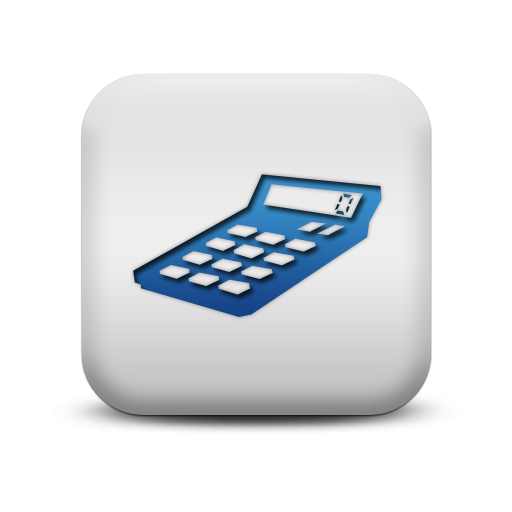Are there parts of the program application where providing in-form calculations would increase the accuracy of data inputs (like showing an applicant their long-term average PV energy output)?

Are there Data Fields that need to be calculated or created for certain Reports or Dashboards?

Which Forms do require calculations that can be handled by Formulas?
Should a Formula result trigger an Automation?

##### Locating the Formulas featureFigure 1: PROGRAM DESIGN >> Formulas

##### How to work with Formulas

The Formulas feature has been designed to enable math to be performed in Forms automatically by PowerClerk using references to Data Fields and constants. An intuitive drag-and-drop Formula editor is used to configure Formulas.

Formulas can perform numeric operations (addition, subtraction, multiplication, division) as well as Boolean operations (greater than, less than, is equal to, is same as, is not same as, etc.) on numeric data fields, and can also perform Boolean operations (is equal to, is not) on Boolean data fields (true/false) on non-numeric fields. A few examples have been provided in Figure 2 and Figure 3 below.Figure 2: Example Numeric FormulaFigure 3: Example Boolean Formula

Once a Formula has been saved, you can create a Calculated Field to use this Field in your Forms. In the example in Figure 4 below, the Calculated Field created from the Formula comparing Estimated Annual Production to Historical Annual Consumption (kWh) designed in Figure 3 above populates the result automatically in the Form and updates automatically as data field values change.Figure 4: Calculated Field element in a Form

Formulas can also be used within Automations to trigger Action Rules as shown in Figure 5 below:Figure 5: Formulas in Automations

### Formula Data Dictionary

##### Supported Data Types (Input):
Color Data Type ReferenceBlue accepts Numeric inputs Numeric constant or Data Field Reference types (integer, decimal, price)Orange accepts Boolean inputs True, False, (Multiple) Choice, Drop-down List, Attachment Approval/Rejection or Data Field references types which return a True/False result (Checkbox, Boolean Calculated Field Result)Green accepts Text inputs Text String or Text Data Field reference types (Single Line Text, Paragraph Text, Email, or Contact Data Fields)Purple accepts Date inputs Date or Date Field referenceBlack accepts Dynamic inputs Formula reference types (Numeric, Boolean/Relational, Text, Date)
Additionally, inputs can also accept formula operations that match their respective data types.

##### Supported Formula Operations (Output):
Numeric Operations
(Returns a Numeric result)
Operator Name DescriptionAddition Adds two valuesSubtraction Subtracts two valuesMultiplication Multiplies two valuesDivision Divides two valuesContant Can contain any Numeric valueCan reference any Numeric Data FieldCompares sets of data and tests the results:
If the results are true, the THEN instructions are taken
If not, the ELSE instructions are taken e.g. If (A = TRUE), Then B, Else CMin Returns the smallest value from the two Numeric values provided e.g. (1,2) = 1Max Returns the largest value from the two Numeric values provided e.g. (1,2) = 2Exponent Represents how many times a Numeric value is multiplied by itself
e.g. (2^5) = 2 x 2 x 2 x 2 x 2 = 32Log e.g. Log (1000) = 3 (10 x 10 x 10 = 10^3 = 1,000)

Relational Formula Operations
(Subset of Boolean Formula Operations that tests a relationship and returns a True/False result)
Operator Name DescriptionEqual To Accepts a Numeric or Text inputNot Equal To Accepts a Numeric or Text input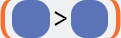Greater Than Accepts a Numeric inputLess Than Accepts a Numeric input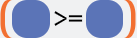Greater Than or Equal To Accepts a Numeric inputLess Than or Equal To Accepts a Numeric inputIs Before/After Accepts a Date input

Boolean Formula Operations
(Returns a True/False result)
Operator Name Description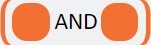And True if and only if both conditions are true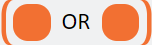Or True if either condition is true (or if both are true)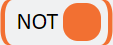Not Changes true to false, and false to trueTrue Constant of trueFalse Constant of false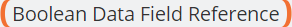References a Checkbox Data Field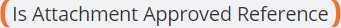Determines if an Attachment is approved by an administratorDetermines if an Attachment is rejected by an administratorReferences a Multiple Choice Data Field & looks to see if the Data Field is equal to the designated choice in the argumentReferences a Multiple Choice Data Field & looks for if the Data Field is not equal to the designated choice in the argument

Text Formula Operations
(Returns a Text result except for the Length of Operation, which returns a Numeric result)
Operator DescriptionReferences any Text value enteredReferences Single Line Text, Paragraph Text, Email, or Contact Data FieldsCompares Boolean True/False data and tests the Text results
If the results are true, the THEN instructions are taken
If not, the ELSE instructions are taken e.g. If (A = TRUE), Then B, Else C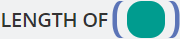Determines the length of a given Text value and returns a Numeric value
e.g. Length of (PowerClerk) = 10Combines two Text values and returns a concatenated Text string
e.g. “foo” + “bar” = “foobar”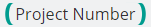Enables referencing the respective project number (e.g. “CPR-00123”) within a FormulaReference non-string Data Field types such as Numeric, Boolean,
Choice, and Date Data Fields as text representations enabling usage
of non-string Data Fields in context of any of the other Text Formula
Operators (e.g. IF/THEN/ELSE, LENGTH OF(), CONCATENATE)

Data Formula Operations
(Returns a Date result)
Operator Description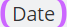References a designated DateReferences a Date Data FieldReferences a Numeric value before/after a Date or Date Data Field reference e.g. (7) days after (Date)

Formula Reference Operations
(Returns a result based on the primary operation)
Operator Description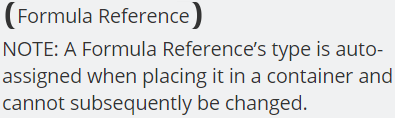Place a Formula reference within any other Formula
operator to avoid having to create multiple
layers of complex Formulas:Multi-Instance Formula Operators
(valdation of multi-instance fields to ensure all field instances adhere to the desired criteria)
Operator DescriptionReturns Boolean True if all instance values across the entire multi-instance field match, or False if not.If all values across the multi-instance field match, returns that exact single value (whether that value is numerical, text, Boolean, or date).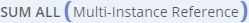Returns the summation of all values across a multi-instance field of numerical data type.

##### Dynamic Formula References

Use Dynamic Formula References to simplify complex Formula expressions by referencing existing Formulas as components (or Formula segments) of the larger Formula.

To reference a Formula within another Formula, please use the Formula Reference operand that is listed under the “Dynamic” section in the Formula editor:Figure 6: Dynamic Formula References

The Dynamic Formula Reference operator itself is not designated to return a Numerical, Boolean, Text, or Date result. Once a Dynamic Formula Reference operator is placed into a Formula container, the operator will turn into the respective data type. Then, a list of the available Formula Reference Expressions (matching the respective data type) will be displayed under the Properties tab on the right-hand side of the editor.Figure 7a: Adding a Formula Reference determines data typeFigure 7b: Dynamic Formula Reference data types

##### Rules of Formula References
1. Incomplete Formulas cannot be referenced.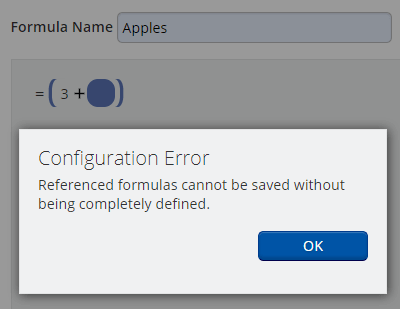Figure 8a: Formula Reference Rule 1

2. You cannot create circular Formula References.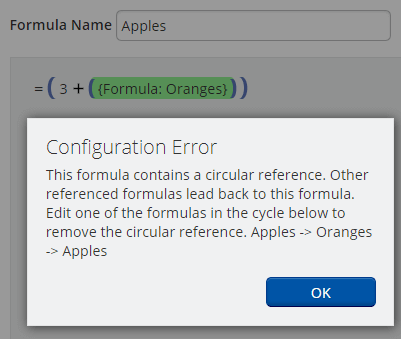Figure 8b: Formula Reference Rule 2

3. A Formula Reference cannot be the only component of a Formula.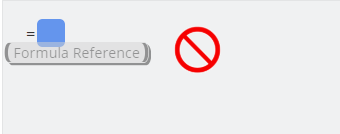Figure 8c: Formula Reference Rule 3

Completed Formulas that incorporate Dynamic Formula References may be used to create Calculated Fields. Please note: Once the Calculated Field has been created, any Formula Reference is fully resolved into the Calculated Field and indistinguishable from other Formula components.

When the Calculated Field is included on a Form, and configured to “Show Details” (as shown in Figure 9), each individual component of the Formula will be displayed as any prior Formula References have been flattened into the Calculated Field.Figure 9a: Formula showing the Formula ReferenceFigure 9b: Calculated Field with a flattened Formula ReferenceFigure 9c: Calculated Field as displayed on a Form without any Formula References any longer

##### Calculated Fields and Advanced Visibility Rules

One of the great advantages of Calculated Fields are that you can use them within your Forms without having to display the actual Field result on the Form any longer. In addition you can also reference Formulas to define Advanced Visibility Rules as shown in Figure 10: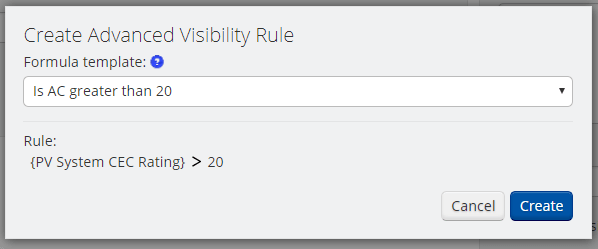Formulas can also reference Calculated Fields. Currently only one level of reference is supported (i.e. you cannot reference Calculated Fields that reference other Calculated Fields).

### Video Guides

##### Build A Formula

This video guide will demonstrate how to build a formula through our visual design surface. Use PowerClerk to calculate key data fields that streamline your administrator workflow.

##### Automation with Formulas in Action Rules

The following video will present you the concept of triggering Automations based on a Formula’s result:

##### Formulas and Advanced Visibility Rules

To control the Conditional Visibility of From fields you can use Formula logic to adapt the display of your Forms to a vast variety of business scenarios:

##### Calculated Fields

Display the results of Formula calculations on Forms, Communications, Document Templates, and Reports. This video demonstrates how to use Calculated Fields within your program:

A full list of all Video Guides can also be found here.

### FAQs

Q: What should I keep in mind when working with Formulas?
A: Please consider the following guidance when working with Formulas:
• Formulas cannot reference another formula.
• The Calculated Fields are dynamic. If any field included in the calculation changes, the Calculated Result field will update accordingly.
• Calculated Fields cannot be read-only (they don’t need to be, as they are calculated based off other form fields).
Q: I am looking for a tutorial on how to get started with Formulas, can you help?
A: Please consider the following instructions as an exercise for your sandbox environment to familiarize yourself with PowerClerk's Formula functionality. With that said, let's get started!
• STEP 1: In your sandbox environment create a form by clicking Program Design >> Forms >> New Form. Name this new form "Calculator". Add two Integer fields (labelled "Value A" and "Value B" below) and one Multiple Choice field (labelled "Operators") with the following choices as shown below:Save your new form by clicking on "Save".
• STEP 2: Navigate now to the Formula menu by clicking on Program Design >> Formulas >> New Formula and name this new Formula "MyCalculator"
• STEP 3: Drag each of the available operator fields onto the scratchpad as shown below:• STEP 4: Drag the (Data Field Reference) data type into each of the blue operator options and assign Value A and Value B as shown below and finish the other operator blocks accordingly: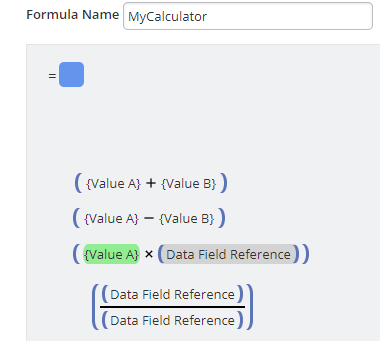• STEP 5a: For ADDITIONS: Drag a (IF __ THEN __ ELSE __ ) data type onto the scratch pad as well as one of the Boolean operators named ( Choice Data Field Reference == Choice ):STEP 5b: Drag the data types into their positions as indicated below:Please notice how the Boolean operator could only be placed into the orange position within the (IF __ THEN __ ELSE __ ) data type.
• STEP 6a: Repeat these steps for SUBTRACTION, MULTIPLICATION, and DIVISION operator blocks - your scratchpad should look now similar to this screenshot below:STEP 6b: Click now on each of the ( Choice Data Field Reference == Choice ) data type and assign as value "Operators" and choose the respective Choice option (i.e. "+ addition" in the screenshot below):STEP 6c: Repeat this procedure for all other operators and your scratchpad should now look similar to this:• STEP 7: Now drag the DIVISION formula block into the open blue position of the MULTIPLICATION formula block and repeat these steps until your scratchpad looks like this (Please note for the remaining blue position within the DIVISION formula block you assign data type (constant) and give it a value of 0):• STEP 8: Save your formula.
• STEP 9: In the Formula overview screen select your "MyCalculator" Formula and click on the Create Calculated Field button - you are now ready to use this Calculated Field within your original Form:• STEP 10: Click now on your original Form in Program Design >> Forms and click the "Edit Form" button:• STEP 11: Drag a "Calculated Field Results" onto your form as shown below:• STEP 12: Select "MyCalculator" as the Formula and designate the visibility to be conditional on the Operators field not to be empty:• STEP 13: Save your form now.
• STEP 14: We now need to configure your form to display it on the HOME screen. Click on Program Design >> Forms and select your original form and click this time on "Configure Form". In the following screen select option "Use for New Project button" and hit "Save":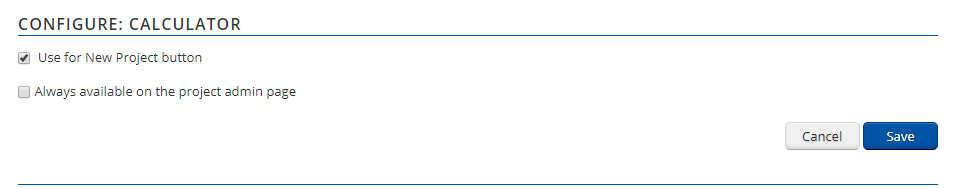• CONGRATULATIONS! Click now on HOME >>to enjoy your new calculator:Q: What is the template tag syntax for a calculated field?
A: Calculated fields are listed in the Formulas > Calculated Fields section, but their template tags can be found in PowerClerk's Data Fields functionality as with any other data field. You can search for your Calculated Field and copy the accompanying template tag as exemplified below for `{data: My Calculated Field}`: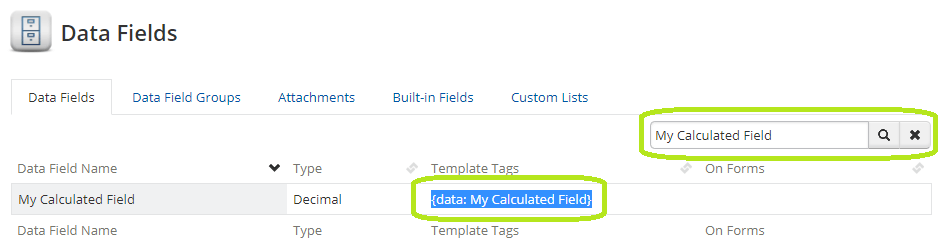Copying a Calculated Field's Template Tag